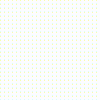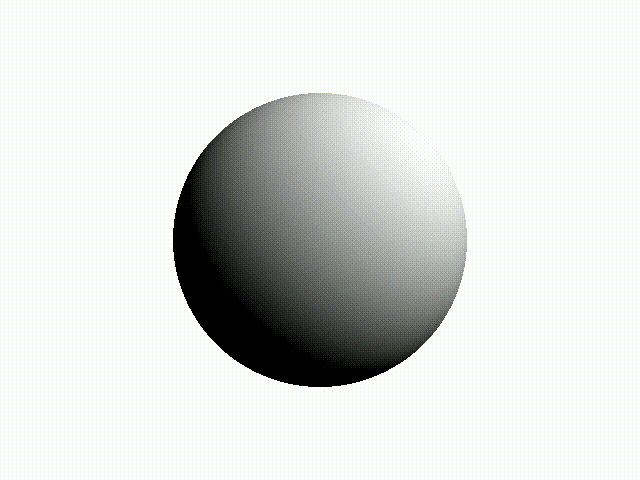Fork me on GitHub
Source file: literate-video.fut

# Generating videos with literate Futhark

A video is just a collection of images called frames. However, for memory reasons it is usually best not to generate all the frames simultaneously. Therefore the `:video` command is a bit more intricate than `:img`. To generate a video, we must define a frame function that takes as input a state and returns a frame and a new state value.

First we define a function for converting a light intensity into an `u32` encoding an ARGB colour.

``````def grey (light: f32) : u32 =
let x = u32.f32(255 * f32.min 1 (f32.max 0 light))
in (x << 16) | (x << 8) | x``````

Then we define a frame function where some of the parameters will be partially applied. The state parameter `t` represents the current time, and is increased by the time delta `td` for every frame.

``````entry frame (width: i64) (height: i64) (td: f32) (t: f32): ([height][width]u32, f32) =
(replicate height (replicate width (grey(0.5+f32.cos(t*10)/2))),
t + td)``````
``````> :video (frame 100i64 100i64 0.02f32, 0f32, 314i64);
fps: 24
format: gif``````That is not terribly exciting. Let’s try something more interesting - specifically, a simple ray marcher that renders a blobby sphere. First, we’ll need to do some vector calculations, so we’ll import code from another example.

``````module vectors = import "3d-vectors"

module vec3 = vectors.mk_vspace_3d f32
type vec3 = vec3.vector``````

Next we’ll define a function for converting a point on a sphere into latitude and longtitude (UV mapping):

``````def uv (p: vec3) : (f32,f32) =
let d = vec3.normalise p
in (0.5 + f32.atan2 d.x d.z / (2*f32.pi),
0.5 + f32.asin d.y / f32.pi)``````

Now we can define a function for determining the radius of a blobby sphere at a given point.

``````def radius_at (t: f32) (p: vec3) : f32 =
let (u,v) = uv p
in (1+f32.sin(u*20*f32.pi+t)*f32.sin(t))/2 +
(1+f32.cos(v*20*f32.pi+t)*f32.sin(t))/2``````

The signed distance function is now trivial.

``````def sdf (t: f32) (p: vec3) : f32 =
vec3.length p - radius_at t p``````

To trace the sphere, we perform ray marching into the scene, with up to 128 steps. If we make it to 128, we assume a miss. The logic is a bit convoluted due to lack of recursive functions.

``````type hit = #hit vec3 | #miss

def trace t (orig: vec3) (dir: vec3) : hit =
let not_done (i, _) = i < 128
let march (i, pos) =
let d = sdf t pos
in if d < 0
then (1337, pos)
else (i + 1, pos vec3.+ ((f32.max (d*0.1) 0.01) `vec3.scale` dir))
in iterate_while not_done march (0,orig)
|> \(i, hit) -> if i == 1337 then #hit hit else #miss``````

Finally, we’ll need a way to compute a surface normal for lighting. This can be done with a single invocation of reverse-mode automatic differentiation.

``````def grad f x = vjp f x 1f32

def distance_field_normal t pos =

This concludes the actual geometry code. Now we just have to construct camera rays.

``````def camera_ray width height i j =
let fov = f32.pi/3
let x = (f32.i64 i + 0.5) - f32.i64 width/2
let y = -(f32.i64 j + 0.5) + f32.i64 height/2
let z = -(f32.i64 height)/(2*f32.tan(fov/2))
in vec3.normalise {x,y,z}``````

The actual frame function is quite straightforward - for each pixel generate a ray and see if it collides with the blobby sphere. We use the surface normal to reflect lighting from a light sourse at (10,10,10).

``````entry blob (width: i64) (height: i64) (td: f32) (t: f32): ([height][width]u32, f32) =
let f j i =
let dir = camera_ray width height i j
in match trace t {x=0, y=0, z=3} dir
case #miss ->
0xFFFFFF
case #hit hit ->
let light_dir = vec3.normalise ({x=10, y=10, z=10} vec3.- hit)
let light_intensity = light_dir `vec3.dot` distance_field_normal t hit
in grey light_intensity
in (tabulate_2d height width f, t + td)``````

Finally we can view our sphere it in all its moderately pixelated glory.

``````> :video (blob 640i64 480i64 0.0314f32, 0f32, 100i64);
fps: 24
format: gif``````